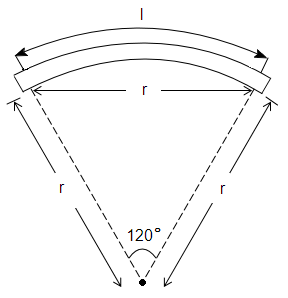# NEET Questions Solved

A bar magnet of length l and magnetic dipole moment M is bent to form an arc which subtends an angle of $120°$ at centre. The new magnetic dipole moment will be

1.  $\frac{3\mathrm{M}}{2\mathrm{\pi }}$

2.  $\frac{3\sqrt{3}\mathrm{M}}{2\mathrm{\pi }}$

3.  $\frac{3\mathrm{M}}{\mathrm{\pi }}$

4.  $\frac{2\mathrm{M}}{\mathrm{\pi }}$Difficulty Level:

• 23%
• 46%
• 18%
• 15%